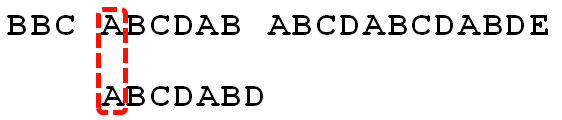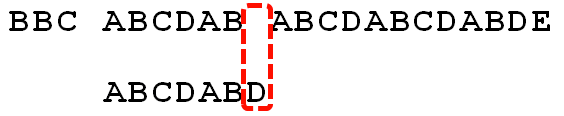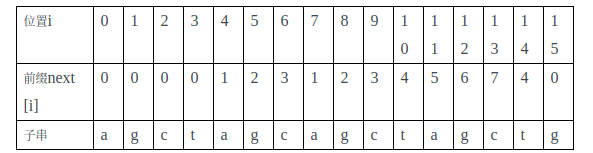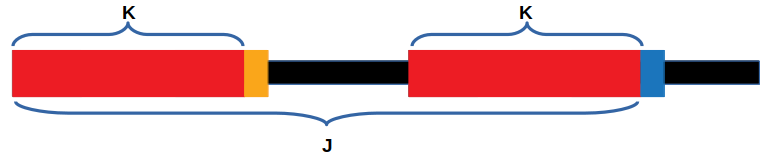KMP算法

0
0
01. 云栖社区>
2. 博客>
3. 正文

KMP算法

colleen 2018-08-31 21:35:00 浏览395

KMPKMP可以在匹配过程中失配的情况下，有效地多往后面跳几个字符，加快匹配速度。• 当前字符匹配成功（即S[i] == P[j]，图一），都令k++j++，继续匹配下一个字符；
• 当前字符匹配失败（即S[i] != P[j]，图二），则令 k不变，j = next[j]。此举意味着失配时，模式串P相对于文本串S向右移动了j - next [j]位。

next数组（前缀数组）• 红色：模式串P当前已经匹配好的相同前后缀
• 蓝色：模式串P当前匹配的位置，就是j
• 橙色：模式串P当前匹配的最长前缀的后一位，即为k• 灰色：当前已经匹配好相同前后缀中的最长公共前后缀
• 紫色：当前已经匹配好相同前后缀中的前缀的后一位

代码

//优化过后的next 数组求法
void GetNextval(char* p, int next[]) {
int pLen = strlen(p);
next = -1;
int k = -1;
int j = 0;
while (j < pLen - 1){
//p[k]表示前缀，p[j]表示后缀
if (k == -1 || p[j] == p[k]){
++j;
++k;
//较之前next数组求法，改动在下面4行
if (p[j] != p[k])
next[j] = k;   //之前只有这一行
else
//因为不能出现p[j] = p[ next[j ]]，所以当出现时需要继续递归，k = next[k] = next[next[k]]
next[j] = next[k];
}
else{
k = next[k];
}
}
}

colleen
+ 关注